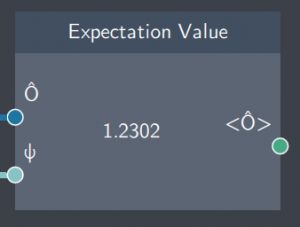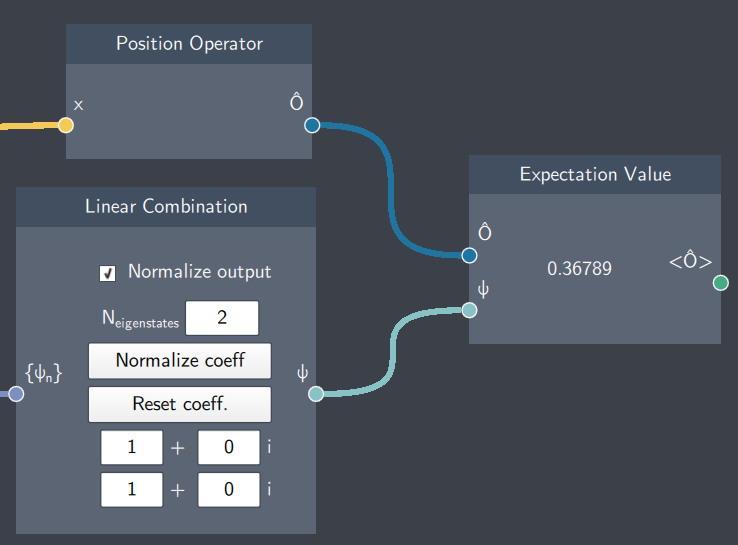# Expectation Value

## Description

This node calculates the expectation value of any operator with regard to an arbitrary state.

For an operator $\hat{O}$ and a state $\psi$, this node calculates $\langle \psi | \hat{O} | \psi \rangle$.## Inputs

The node has the following inputs:

• Operator ($\hat{O}$): Any operator defined in the Operator node such as $\hat{x}$, $\hat{p}$ or $\hat{H}$.
• State ($\psi$): The state for which the expectation value should be calculated.

## Content

The node displays the expectation value of the operator.

## Output

• Expectation Value ($\langle \hat{O} \rangle$): This node outputs the expectation value of the operator ($\hat{O}$) which is provided in the input.

## Example

In the example below, the expectation value of the position operator is calculated with regard to a non-stationary state and displayed in the node.